## 它与随机森林相比如何？

A) 统一初始化样本权重为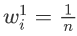.

B) 对于每次迭代 t：

• 找到`ht(x)` 最小化的弱学习 器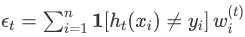.
• 我们根据其准确性为弱学习器设置权重：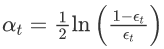• 增加错误分类观察的权重：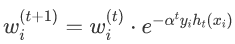.
• 重新归一化权重，使得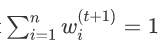.

C）将最终预测作为弱学习器预测的加权多数票：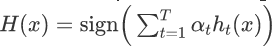.

# 绘图

Boosting集成学习原理与R语言提升回归树BRT预测短鳍鳗分布生态学实例

```def plot(X: np.ndaay,
y: np.ndrry,
cf=None) -> None:
""" 绘制2D的±个样本，可选择决策边界 """

if not ax:
fig, ax = plt.sults(fgsze=(5, 5), di=100)

if saligs is not None:
sies = np.array(spl_wigts) * X.hae\[0\] * 100
else:
sze = np.oes(sape=X.shpe\[0\]) * 100

if cf:
xx, yy = np.ehrid(n.aange(x\_min, x\_max, plot_step),
p.aang(y\_min, y\_max, plot_step))

pdt(np.c_\[xx.ravel(), yy.ravel()\])

# 如果所有的预测都是正类，则相应地调整颜色图。
if list(np.niue(Z)) == \[1\]:
colors = \['r'\]
else:
colors = \['b', 'r'\]

ax.st\_im(in+0.5, \_ax-0.5)
ax.st_lm(ymin+0.5, yax-0.5)```

# 数据集

```def maketat(n: it = 100, rased: it = None):

nclas = int(n/2)

if ranmed:
np.ram.sed(rndoed)

X, y = me\_gainqnes(n=n, n\_fees=2, n_css=2)

plot(X, y)```

# 使用 scikit-learn 进行基准测试

```from skarn.esele import AdosClaser

plat(X, y, bech)

tnr = (prdict(X) != y).man()```

### 随时关注您喜欢的主题

```class AdBst:

def \_\_init\_\_(self):
self.sump = None
self.stup_weght = None
self.erro = None
self.smle_weih = None

def \_ceck\_X_y(self, X, y):
""" 验证关于输入数据格式的假设"""
assrt st(y) == {-1, 1}
reurn X, y```

# 回想一下我们的算法来拟合模型：

• 找到`ht(x)` 最小化的弱学习 器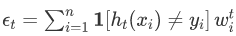.
• 我们根据其准确性为弱学习器设置权重：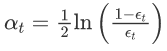• 增加错误分类观察的权重：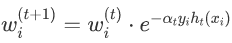. 注意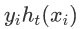当假设与标签一致时将评估为 +1，当它与标签不一致时将评估为 -1。
• 重新归一化权重，使得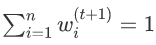.

• 我们在 for 循环之外设置初始统一样本权重，并在每次迭代 t 内设置 t+1 的权重，除非它是最后一次迭代。我们在这里特意在拟合模型上保存一组样本权重，以便我们以后可以在每次迭代时可视化样本权重。
```def ft(slf, X: narry, y: ndray, ites: int):
""" 使用训练数据拟合模型 """

X, y = slf.\_chck\_X_y(X, y)
n = X.shpe\[0\]

# 启动Numpy数组
self.smle_wegts = np.zos(shpe=(itrs, n))
self.tumps = np.zeos(she=iters, dtpe=obect)

# 均匀地初始化权重
sef.sampewegts\[0\] = np.one(shpe=n) / n

for t in range(iters):
# 拟合弱学习器
fit(X, y, smpe\_eght=urrsmle\_igts)

# 从弱学习者的预测中计算出误差和树桩权重
predict(X)
err = cu_seghts\[(pred != y)\].sum()# / n
weiht = np.log((1 - err) / err) / 2

# 更新样本权重
newweis = (
crrawe * np.exp(-sum_wiht * y * tupd)
)

# 如果不是最终迭代，则更新t+1的样本权重
if t+1 < ies:
sef.smpe\_wit\[t+1\] = ne\_saml_wigt

# 保存迭代的结果
sef.sups\[t\] = tump```

# 现在让我们把所有东西放在一起，用与我们的基准测试相同的参数来拟合模型。

```def pedc(self, X):
""" 使用已经拟合的模型进行预测 """
supds = np.aray(\[stp.pect(X) for sump in slf.stps\])
return np.sgn(np.dt(self.tum_whts, sumpreds))```

# 表现

```# 将我们单独定义的函数指定为分类器的方法
AaBt.fit = fit

plot(X, y, clf)

err = (clf.prdc(X) != y).mean()```

## 可视化

• 左栏显示了所选择的 “树桩 “弱学习器，它与ht(x)相对应。
• 右边一栏显示了到目前为止的累积强学习器。 Ht(x)。
• 数据点标记的大小反映了它们的相对权重。在上一次迭代中被错误分类的数据点将被更多地加权，因此在下一次迭代中显得更大。
```def truost(clf, t: int):

nwwghts = clf.suweighs\[:t\]

def plotost(X, y, clf, iters=10):
""" 在每个迭代中绘制出弱学习者和累积强学习者。 """

# 更大的网格
fig, axs = subplos(fisze=(8,ters*3),
nrows=iers,
ncls=2,
shaex=True,
dpi=100)

# 绘制弱学习者
plotot(X, y, cf.\[i\],
saplweghs=clf.saple_wigts\[i\],
aoat=False, a=ax1)

#绘制强学习者
truost(clf, t=i + 1)
pltot(X, y, tun_cf,
weights=smplweih\[i\], ax=ax2)

plt.t_aot()```

## 进一步阅读

Kaizong Ye拓端研究室（TRL）的研究员。

​非常感谢您阅读本文，如需帮助请联系我们！QQ在线咨询

15121130882

0571-63341498

## 关注有关新文章的微信公众号

This will close in 0 seconds